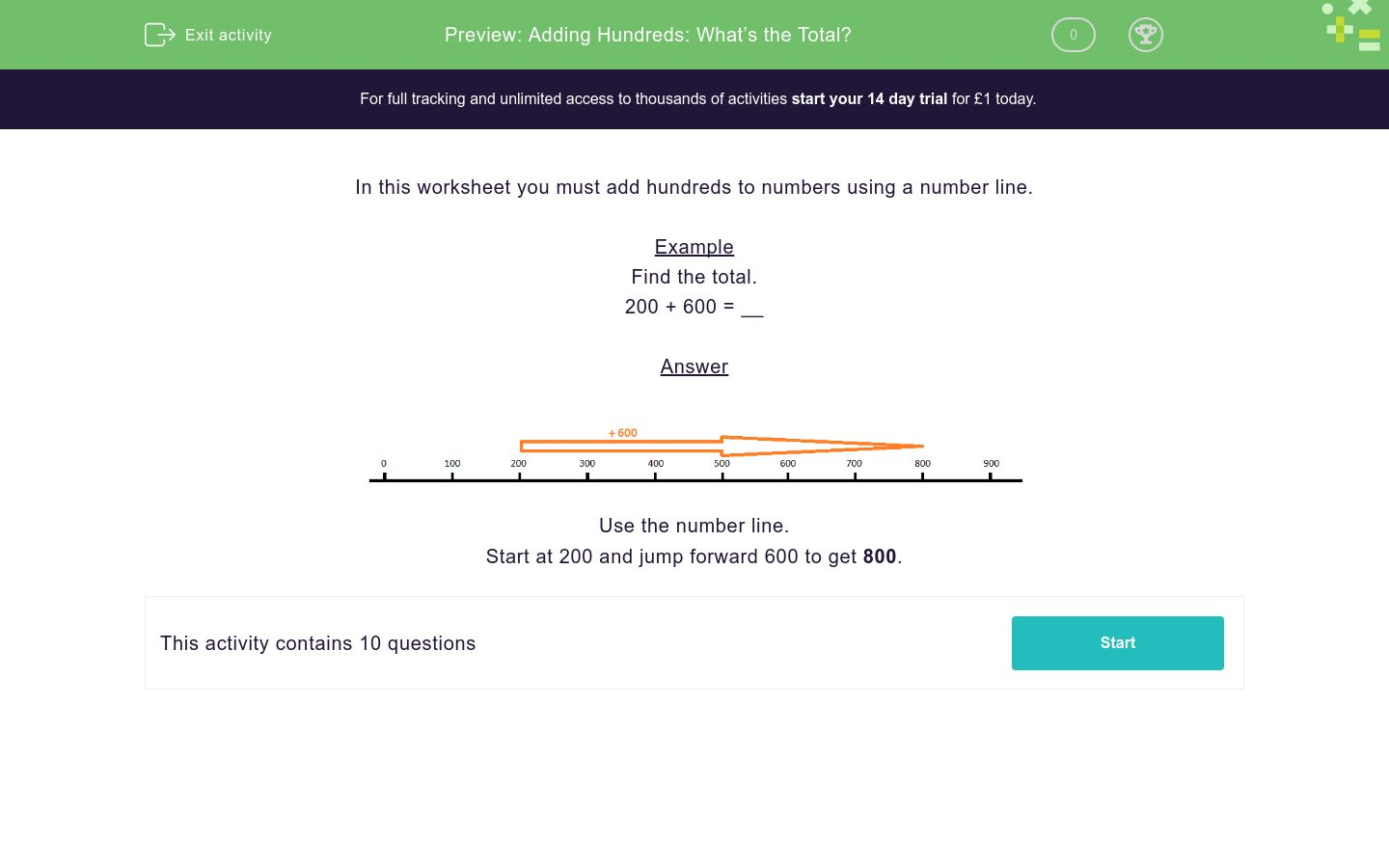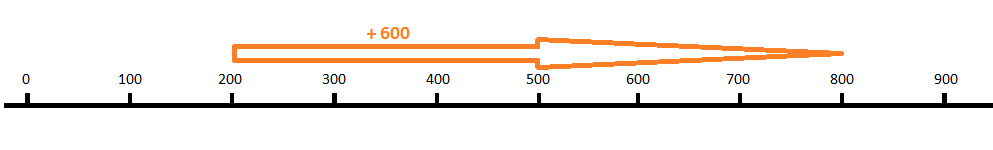# Adding Hundreds: What’s the Total?

In this worksheet, students use a number line to add together two 'hundred' numbers.Key stage:  KS 2

Curriculum topic:   Number: Addition and Subtraction

Curriculum subtopic:   Use Correct Methods to Problem Solve

Difficulty level:### QUESTION 1 of 10

In this worksheet you must add hundreds to numbers using a number line.

Example

Find the total.

200 + 600 = __Use the number line.

Start at 200 and jump forward 600 to get 800.

Find the total.

900 + 300 = __

Find the total.

200 + 300 = __

Find the total.

700 + 700 = __

Find the total.

800 + 500 = __

Find the total.

900 + 600 = __

Find the total.

500 + 700 = __

Find the total.

500 + 800 = __

Find the total.

600 + 400 = __

Find the total.

100 + 600 = __

Find the total.

600 + 300 = __

• Question 1

Find the total.

900 + 300 = __

1200
• Question 2

Find the total.

200 + 300 = __

500
• Question 3

Find the total.

700 + 700 = __

1400
• Question 4

Find the total.

800 + 500 = __

1300
• Question 5

Find the total.

900 + 600 = __

1500
• Question 6

Find the total.

500 + 700 = __

1200
• Question 7

Find the total.

500 + 800 = __

1300
• Question 8

Find the total.

600 + 400 = __

1000
• Question 9

Find the total.

100 + 600 = __

700
• Question 10

Find the total.

600 + 300 = __